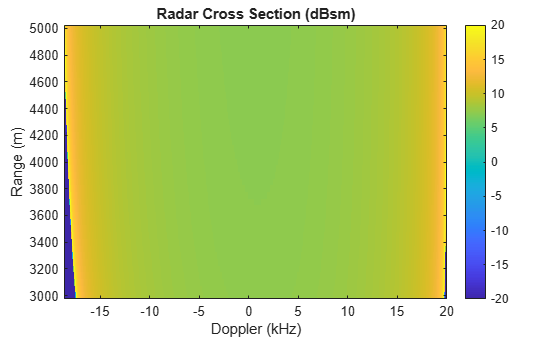# clutterSurfaceRangeDopplerRCS

Surface RCS as a function of range and Doppler

Since R2022b

## Syntax

``rcs = clutterSurfaceRangeDopplerRCS(nrcs,rbins,freq,dopres,alt,speed,dive)``
``rcs = clutterSurfaceRangeDopplerRCS(___,PropagationSpeed=c)``
``rcs = clutterSurfaceRangeDopplerRCS(___,NumDopplerBins=ndop)``
``rcs = clutterSurfaceRangeDopplerRCS(___,NumIntegrationPoints=nri)``
``[rcs,dopbins] = clutterSurfaceRangeDopplerRCS(___)``

## Description

example

````rcs = clutterSurfaceRangeDopplerRCS(nrcs,rbins,freq,dopres,alt,speed,dive)` returns the radar cross-section `rcs` of a surface illuminated by monostatic radar, where `nrcs` – normalized radar cross-section`rbins` – range bin centers`freq` – radar frequency`alt` – radar altitude`dopres` – Doppler resolution`speed` – radar speed`dive` – radar dive angle are the input arguments.```
````rcs = clutterSurfaceRangeDopplerRCS(___,PropagationSpeed=c)` also specifies the signal propagation speed `c`.```
````rcs = clutterSurfaceRangeDopplerRCS(___,NumDopplerBins=ndop)` also specifies the number of Doppler bins, `ndop`. Using this parameter enables Doppler wrapping and makes the clutter output DC-centered.```
````rcs = clutterSurfaceRangeDopplerRCS(___,NumIntegrationPoints=nri)` also specifies the number of points per range bin `nri` used for numerical integration of the reflectivity.```

example

````[rcs,dopbins] = clutterSurfaceRangeDopplerRCS(___)` also returns the Doppler-shift bin values `dopbins`. Units are in Hz.```

## Examples

collapse all

Calculate clutter RCS in a set of range-Doppler cells. The range swath begins at 3000 m and extends to 5000 m with a 50 m range bin width. The radar center frequency is 30 GHz and with a Doppler resolution of 100 Hz. The radar travels at an altitude of 1000 m and with a speed of 100 m/s with a${10}^{\circ }$ dive angle.

```rngbins= 3000:50:5000; freq = 30e9; doplrres = 100; rdralt = 1000; rdrspeed = 100; dive = 10;```

Use a constant-gamma flatland reflectivity model to get the normalized radar cross-section at each range bin. Then compute the grazing angle using the `grazingang` function. Compute the normalized surface reflectivity.

```gamma = surfacegamma('Flatland'); refl = surfaceReflectivityLand( ... 'Model','ConstantGamma','Gamma',gamma); graze = grazingang(rdralt,rngbins,'Model','Flat'); nrcs = refl(graze,freq);```

Calculate and display the radar cross-section of the clutter.

```[rcs,dop] = clutterSurfaceRangeDopplerRCS( ... nrcs,rngbins,freq,doplrres,rdralt, ... rdrspeed,dive); rcs(rcs < 10^-2) = 10^-2; imagesc(dop/1000.0,rngbins,10*log10(rcs)) title('Radar Cross Section (dBsm)') xlabel('Doppler (kHz)') ylabel('Range (m)') axis('xy') colorbar```## Input Arguments

collapse all

Normalized radar cross section of the surface, specified as a length-N nonnegative vector. Each entry in `nrcs` corresponds to a range specified in `rbins`. Units are dimensionless but often expressed as m²/m².

Data Types: `double`

Range bin centers, specified as a real-valued length-N vector. Elements of `rbins` must appear in increasing order and must have at least two elements. The total range swath starts below the first element of `rbins` and extends beyond the last element of `rbins` by half the range bin width. The starting and ending ranges are extrapolated from the first and last bins. Range bins need not be uniformly spaced. No range wrapping due to ambiguous range is performed. Units are in meters.

Example: `[20 25 30 35]`

Data Types: `double`

Radar frequency, specified as a positive scalar. Units are in Hz.

Data Types: `double`

Doppler resolution, specified as a positive scalar. By default, no wrapping is performed in Doppler space. Doppler bins will cover the full Doppler spectrum of clutter at the specified resolution. Units are in Hz.

Example: `50`

Data Types: `double`

Radar altitude, specified as a non-negative scalar. Units are in meters.

Data Types: `double`

Radar speed, specified as a non-negative scalar. Units are in meters/sec.

Example: 50

Data Types: `double`

Radar dive angle, specified as a scalar between –90° and 90°. The dive angle is the angle that the radar velocity vector makes with the horizontal plane. A positive dive angle indicates that the velocity vector is pointing down. Units are in degrees.

Data Types: `double`

Number of dc-centered Doppler bins, specified as a positive integer. Specifying this parameter enables Doppler wrapping. Use this argument with the `NumDopplerBins` name-value pair.

Example: `NumDopplerBins=128`

Data Types: `double`

Signal propagation speed, specified as a positive scalar. Use this argument with the `PropagationSpeed` name-value pair. The default propagation speed is the value obtained from `physconst('LightSpeed')`. Units are in meters/second.

Example: `PropagationSpeed=3e8`

Data Types: `double`

Number of integration points per range bin, specified as a positive integer. Use this argument with the `NumIntegrationPoints` name-value pair.

Example: `NumIntegrationPoints=100`

Data Types: `double`

## Output Arguments

collapse all

Radar cross section, returned as an complex-valued N-by-M matrix where RCS(i,j) gives the RCS of surface clutter in the range-Doppler cell at the ith range and jth Doppler bin. Units are in m².

Doppler bins, returned as length-M vector. By default, the Doppler bins extend over the entire Doppler spectrum of clutter at the resolution specified by `dopres`.

## Version History

Introduced in R2022b